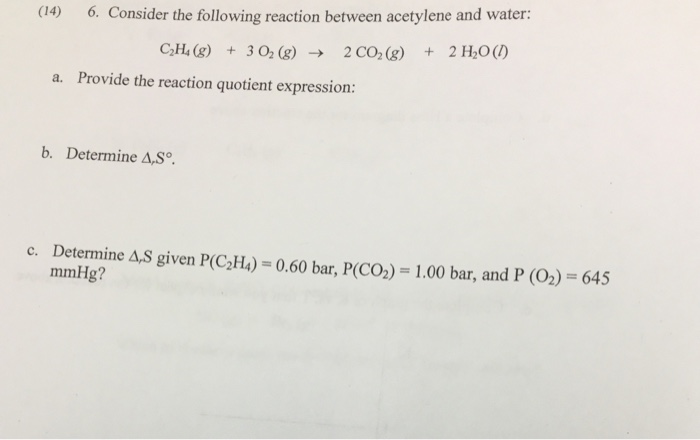# Question & Answer: Consider the following reaction between acetylene and water: C_2 H_4 (g) + 3 O_2 (g)…..Consider the following reaction between acetylene and water: C_2 H_4 (g) + 3 O_2 (g) rightarrow 2 CO_2 (g) + 2 H_2 O(l) a. Provide the reaction quotient expression: b. Determine delta_r S degree. c. Determine delta_r S given P(C_2 H_4) = 0.60 bar, P(CO_2) = 1.00 bar, and P (O_2) = 645 mmHg?